# TypeError: < not supported between instances of str and intLast updated: Feb 16, 2023
12 minMake sure to click on the subheading that covers your error message.

## #TypeError: < not supported between instances of str and int

The Python "TypeError: '<' not supported between instances of 'str' and 'int'" occurs when we use a comparison operator between values of type `str` and `int`.

To solve the error, convert the string to an integer before comparing the values.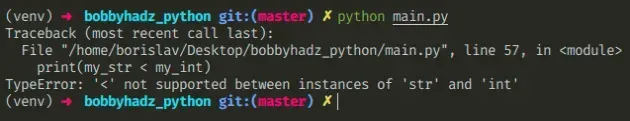## #Using a comparison operator between a string and an integer

Here is an example of how the error occurs.

main.py
```Copied!```my_str = '10'

my_int = 5

# ⛔️ TypeError: '<' not supported between instances of 'str' and 'int'
print(my_str < my_int)
``````

We used a comparison operator between values of incompatible types (str and int) which caused the error.

## #Convert the string to an integer to solve the error

To solve the error, make sure the values you are comparing are compatible types.

main.py
```Copied!```my_str = '10'

my_int = 5

# ✅ convert str to int
print(int(my_str) < my_int)  # 👉️ False

if int(my_str) < my_int:
print('my_str < my_int')
else:
# 👇️ this runs
print('my_str >= my_int')
``````

We used the int() class to convert the string to an integer.

You can also use the float() class if you need to convert the string to a floating-point number.

main.py
```Copied!```my_str = '5.5'

my_int = 5

print(float(my_str) < my_int)  # 👉️ False
``````

We used the `float()` class to convert the string to a floating-point number to be able to compare it to the integer.

Floats and integers are compatible types, so everything works as expected.

You can also use the `str()` class if you meant to compare 2 strings instead of 2 numbers.

## #The `input()` function always returns a string

If you use the `input()` built-in function, all of the values the user enters get converted to strings (even numeric values).

main.py
```Copied!```from_input = input('Enter your fav number: ')
print(from_input)  # 👉️ 3
print(type(from_input))  # 👉️ <class 'str'>

my_int = 5

# ⛔️ TypeError: '<=' not supported between instances of 'str' and 'int'
print(from_input <= my_int)
``````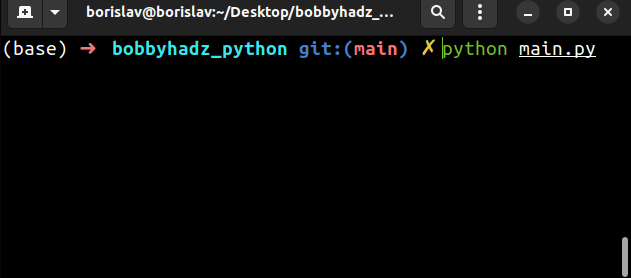The input function converts the data to a string and returns it.

Even if the user enters a number, it gets converted to a string before it is returned from `input()`.

To solve the error, pass the string to the `int()` (or `float()`) class.

main.py
```Copied!```from_input = input('Enter your fav number: ')
print(from_input)  # 👉️ 3
print(type(from_input))  # 👉️ <class 'str'>

my_int = 5

print(int(from_input) <= my_int)  # 👉️ True

if int(from_input) <= my_int:
print('success')
``````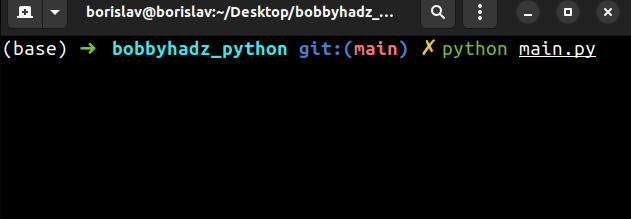We converted the string from the `input` function back to an integer and compared the two numbers.

You can use a try/except statement if you need to handle the error if the user enters an invalid integer.

main.py
```Copied!```try:
from_input = input('Enter your fav number: ')
print(from_input)  # 👉️ "abc"
print(type(from_input))  # 👉️ <class 'str'>

my_int = 5

print(int(from_input) <= my_int)
except ValueError:
print('Invalid integer supplied')
``````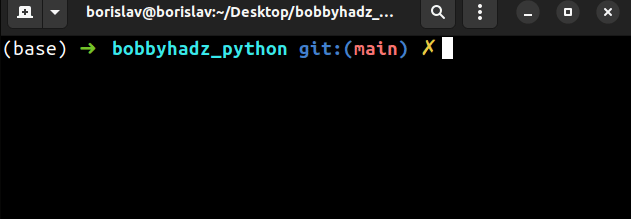The values on the left-hand and right-hand sides of the comparison operator need to be of compatible types.

## #Calling the `min()` and `max()` functions with an int and a string

The error also occurs when you call the `min()` and max() functions with an integer and a string.

main.py
```Copied!```num_1 = 15
num_2 = '20'  # 👈️ stores a string

# ⛔️ TypeError: '<' not supported between instances of 'str' and 'int'
print(min([num_1, num_2]))

# ⛔️ TypeError: '>' not supported between instances of 'int' and 'str'
print(max([num_2, num_1]))
``````

The `num_2` variable stores a string, so it can't be compared to an integer.

We can convert the value to an integer to solve the error.

main.py
```Copied!```num_1 = 15
num_2 = '20'  # 👈️ stores a string

print(min([num_1, int(num_2)])) # 👉️ 15

print(max([int(num_2), num_1])) # 👉️ 20
``````

If you need to convert all values in a list to integers, use a list comprehension.

main.py
```Copied!```a_list = ['15', '20', 25, '30']

new_list = [int(x) for x in a_list]
print(new_list) # 👉️ [15, 20, 25, 30]

print(min(new_list)) # 👉️ 15
print(max(new_list)) # 👉️ 30
``````

List comprehensions are used to perform some operation for every element or select a subset of elements that meet a condition.

On each iteration, we convert the current item to an integer and return the result.

## #Checking what type the variable stores

If you aren't sure what type of object a variable stores, use the built-in `type()` class.

main.py
```Copied!```my_str = '13'

print(type(my_str))  # 👉️ <class 'str'>
print(isinstance(my_str, str))  # 👉️ True

my_int = 5

print(type(my_int))  # 👉️ <class 'int'>
print(isinstance(my_int, int))  # 👉️ True
``````

The type class returns the type of an object.

The isinstance function returns `True` if the passed-in object is an instance or a subclass of the passed in class.

Values such as floats and integers are compatible and can be compared. However, you can't compare a string to an integer.

The string has to be converted to an integer using the `int()` class before the comparison.

## #TypeError: < not supported between instances of list and int

The Python "TypeError: '<' not supported between instances of 'list' and 'int'" occurs when we use a comparison operator between values of type `list` and `int`.

To solve the error, access the list at a specific index or compare the list's length to an integer.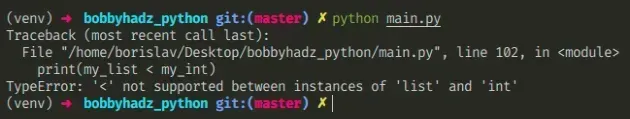Here is an example of how the error occurs.

main.py
```Copied!```my_list = [3, 5, 8]

my_int = 10

# ⛔️ TypeError: '<' not supported between instances of 'list' and 'int'
print(my_list < my_int)
``````

We used a comparison operator between values of incompatible types (list and int) which caused the error.

To solve the error, make sure the values you are comparing are of compatible types.

## #Access the list at a specific index and compare the value

One way to solve the error is to access a specific item in the list.

main.py
```Copied!```my_list = [3, 5, 8]

my_int = 10

print(my_list < my_int)  # 👉️ True

if my_list < my_int:
# 👇️ this runs
print('success')
``````

We accessed the list item at index `0` (the first item) and compared it to an integer.

Python indexes are zero-based, so the first item in a list has an index of `0`, and the last item has an index of `-1` or `len(a_list) - 1`.

main.py
```Copied!```my_list = [3, 5, 8]

print(my_list)  # 👉️ 3
print(my_list)  # 👉️ 5
print(my_list)  # 👉️ 8
``````

## #Make sure the values you are comparing are of compatible types

The values you are comparing have to be of compatible types. If the list contains numbers wrapped in a string, convert the string to a number using the `int()` or `float()` classes.

main.py
```Copied!```my_list = ['3', '5', '8']

my_int = 10

print(int(my_list) < my_int)  # 👉️ True
``````

The list stores strings, so we had to use the `int()` class to convert the string to an integer before the comparison.

## #Filtering the integers in a list based on a comparison

If you meant to filter out integers in a list based on a comparison, use a list comprehension.

main.py
```Copied!```my_list = [3, 5, 8, 10, 15]

new_list = [x for x in my_list if x > 7]

print(new_list)  # 👉️ [8, 10, 15]
``````

The example returns a new list that only contains the values that are greater than `7`.

## #Comparing the list's length to an integer

If you meant to compare the list's length to an integer, use the `len()` function.

main.py
```Copied!```my_list = [3, 5, 8]

my_int = 10

print(len(my_list) > my_int)  # 👉️ False

print(len(my_list))  # 👉️ 3

if len(my_list) < my_int:
# 👇️ this runs
print("The list's length is less than 10")
``````

The len() function returns the length (the number of items) of an object.

The argument the function takes may be a sequence (a string, tuple, list, range or bytes) or a collection (a dictionary, set, or frozen set).

## #Comparing the sum of the items in a list to an integer

If you meant to compare the sum of the items in the list to an integer, use the sum() function.

main.py
```Copied!```my_list = [3, 5, 8]

my_int = 10

print(sum(my_list) > my_int)  # 👉️ True

print(sum(my_list))  # 👉️ 16

if sum(my_list) > my_int:
# 👇️ this runs
print('The sum is greater than 10')
``````

The sum function takes an iterable, sums its items from left to right and returns the total.

The values on the left-hand and right-hand sides of the comparison operator need to be of compatible types.

## #Checking what type a variable stores

If you aren't sure what type of object a variable stores, use the built-in `type()` class.

main.py
```Copied!```my_list = [3, 5, 8]

print(type(my_list))  # 👉️ <class 'list'>
print(isinstance(my_list, list))  # 👉️ True

my_int = 10

print(type(my_int))  # 👉️ <class 'int'>
print(isinstance(my_int, int))  # 👉️ True
``````

The type class returns the type of an object.

The isinstance function returns `True` if the passed-in object is an instance or a subclass of the passed-in class.

## #TypeError > not supported between instances of float and str

The Python "TypeError: '>' not supported between instances of 'float' and 'str'" occurs when we use a comparison operator between values of type `float` and `str`.

To solve the error, convert the string to a float before comparing the values.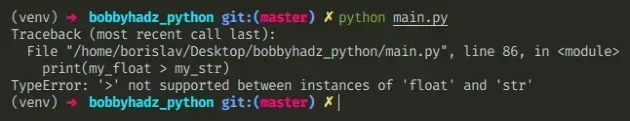Here is an example of how the error occurs.

main.py
```Copied!```my_float = 1.1

my_str = '2.2'

# ⛔️ TypeError: '>' not supported between instances of 'float' and 'str'
print(my_float > my_str)
``````

We used a comparison operator between values of incompatible types (float and str) which caused the error.

## #Convert the string to a float to solve the error

To solve the error, make sure the values you are comparing are of compatible types.

main.py
```Copied!```my_float = 1.1

my_str = '2.2'

# ✅ convert str to float
print(my_float > float(my_str))  # 👉️ False

if my_float > float(my_str):
print('true')
else:
# 👇️ this runs
print('false')
``````

We used the `float()` class to convert the string to a floating-point number. You can also use the `int()` class if you need to convert the string to an integer.

You can also use the `str()` class if you meant to compare 2 strings instead of 2 numbers.

## #Solve the error when using `pandas`

If you use `pandas`, make sure to cast the items in the column to floats.

main.py
```Copied!```import pandas as pd

d = {'col1': ['1.1', '2.2'], 'col2': ['3.3', '4.4']}
df = pd.DataFrame(data=d)

# ✅ cast items to float
df['col1'] = df['col1'].astype(float)

print(3.14 > df['col1']) # 👉️ True
``````

You can also use the `str()` class instead of `float` if you meant to compare 2 strings.

## #The `input()` function always returns a string value

Note that the `input()` built-in function returns a string even if the user provides a floating-point number.

main.py
```Copied!```my_float = 1.1

from_input = input('Enter your fav number: ')
print(from_input)  # 👉️ 2.2
print(type(from_input))  # 👉️ <class 'str'>

# ⛔️ TypeError: '<' not supported between instances of 'float' and 'str'
print(my_float < from_input)
``````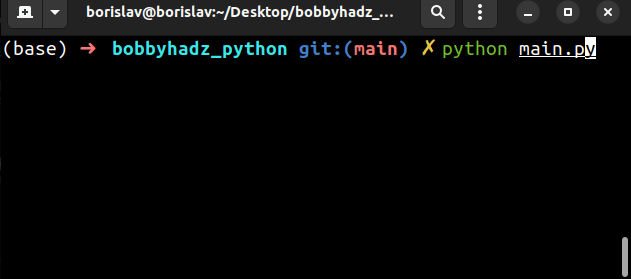The input function converts the data to a string and returns it.

Even if the user enters a number, it gets converted to a string before it is returned from `input()`.

To solve the error, pass the string to the `float()` (or `int()`) class.

main.py
```Copied!```my_float = 1.1

from_input = input('Enter your fav number: ')
print(from_input)  # 👉️ 2.2
print(type(from_input))  # 👉️ <class 'str'>

print(my_float < float(from_input))  # 👉️ True
``````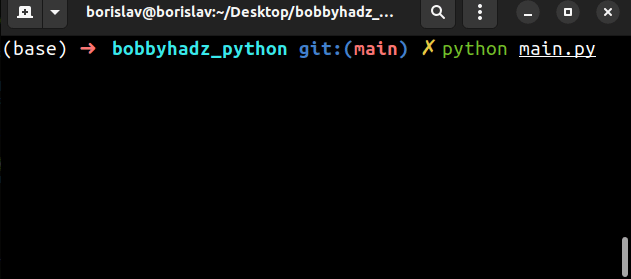We converted the string from the `input` function back to a `float` and compared the two numbers.

You can use a `try/except` statement if you need to handle the error if the user enters an invalid float.

main.py
```Copied!```my_float = 1.1

try:
from_input = input('Enter your fav number: ')
print(from_input)  # 👉️ abc
print(type(from_input))  # 👉️ <class 'str'>

print(my_float < float(from_input))

except ValueError:
# 👇️ this runs
print('Invalid float supplied')
``````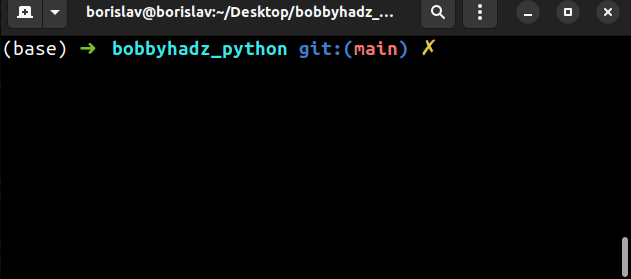The values on the left-hand and right-hand sides of the comparison operator need to be of compatible types.

## #Calling the `min()` and `max()` functions with a float and a string

The error also occurs when you call the `min()` and `max()` functions with a float and a string.

main.py
```Copied!```num_1 = 1.1
num_2 = '2.2'  # 👈️ stores a string

# ⛔️ TypeError: '<' not supported between instances of 'str' and 'float'
print(min([num_1, num_2]))

# ⛔️ TypeError: '>' not supported between instances of 'float' and 'str'
print(max([num_2, num_1]))
``````

The `num_2` variable stores a string, so it can't be compared to a floating-point number.

We can convert the value to a float to solve the error.

main.py
```Copied!```num_1 = 1.1
num_2 = '2.2'  # 👈️ stores a string

print(min([num_1, float(num_2)]))  # 👉️ 1.1

print(max([float(num_2), num_1]))  # 👉️ 2.2
``````

If you need to convert all values in a list to floating-point numbers, use a list comprehension.

main.py
```Copied!```a_list = ['1.1', '2.2', 3.3, '4.4']

new_list = [float(x) for x in a_list]
print(new_list)  # 👉️ [1.1, 2.2, 3.3, 4.4]

print(min(new_list))  # 👉️ 1.1
print(max(new_list))  # 👉️ 4.4
``````

List comprehensions are used to perform some operation for every element or select a subset of elements that meet a condition.

On each iteration, we convert the current item to a float and return the result.

## #Checking what type a variable stores

If you aren't sure what type of object a variable stores, use the built-in `type()` class.

main.py
```Copied!```my_float = 1.1

print(type(my_float))  # 👉️ <class 'float'>
print(isinstance(my_float, float))  # 👉️ True

my_str = '3.14'

print(type(my_str))  # 👉️ <class 'str'>
print(isinstance(my_str, str))  # 👉️ True
``````

The type class returns the type of an object.

The isinstance function returns `True` if the passed-in object is an instance or a subclass of the passed-in class.

## #TypeError > not supported between instances of tuple and int

The Python "TypeError: '>' not supported between instances of 'tuple' and 'int'" occurs when we use a comparison operator between values of type `tuple` and `int`.

To solve the error, access the tuple at a specific index or compare the tuple's length to an integer.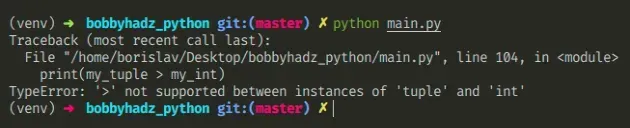Here is an example of how the error occurs.

main.py
```Copied!```my_tuple = 2, 4, 6

my_int = 5

# ⛔️ TypeError: '>' not supported between instances of 'tuple' and 'int'
print(my_tuple > my_int)
``````

We used a comparison operator between values of incompatible types (tuple and int) which caused the error.

To solve the error, make sure the values you are comparing are of compatible types before the comparison.

## #Accessing the tuple at a specific index

One way to solve the error is to access a specific item in the tuple.

main.py
```Copied!```my_tuple = 2, 4, 6

my_int = 5

print(my_tuple > my_int)  # 👉️ False

if my_tuple > my_int:
print('yes')
else:
# 👇️ this runs
print('no')
``````

We accessed the tuple item at index `0` (the first item) and compared it to an integer.

Python indexes are zero-based, so the first item in a tuple has an index of `0`, and the last item has an index of `-1` or `len(my_tuple) - 1`.

If the values in your tuple are of type string, use the `int()` class to convert them to an integer before the comparison.

main.py
```Copied!```my_tuple = ('2', '4', '6')

my_int = 5

print(int(my_tuple) > my_int)  # 👉️ False

if int(my_tuple) > my_int:
print('yes')
else:
# 👇️ this runs
print('no')
``````
The values you are comparing have to be of compatible types. If the tuple contains numbers wrapped in a string, convert the string to a number using the `int()` or `float()` classes.

## #Filtering a tuple based on a comparison

If you meant to filter out integers in a tuple based on a comparison, use a list comprehension.

main.py
```Copied!```my_tuple = 2, 4, 6, 8

my_int = 5

new_tuple = tuple([x for x in my_tuple if x > my_int])

print(new_tuple)  # 👉️ (6, 8)
``````

The example returns a tuple that only contains the elements that are greater than 5.

## #Comparing a tuple's length to an integer

If you meant to compare the tuple's length to an integer, use the `len()` function.

main.py
```Copied!```my_tuple = 2, 4, 6, 8

my_int = 5

print(len(my_tuple) > my_int)  # 👉️ False

print(len(my_tuple))  # 👉️ 4
``````

The len() function returns the length (the number of items) of an object.

The argument the function takes may be a sequence (a string, tuple, list, range or bytes) or a collection (a dictionary, set, or frozen set).

## #How tuples are constructed in Python

In case you declared a tuple by mistake, tuples are constructed in multiple ways:

• Using a pair of parentheses `()` creates an empty tuple
• Using a trailing comma - `a,` or `(a,)`
• Separating items with commas - `a, b` or `(a, b)`
• Using the `tuple()` constructor

## #Compare the sum of the tuple to an integer

If you meant to compare the sum of the items in the tuple to an integer, use the `sum()` function.

main.py
```Copied!```my_tuple = 2, 4, 6, 8

my_int = 5

print(sum(my_tuple) > my_int)  # 👉️ True

print(sum(my_tuple))  # 👉️ 20
``````

The `sum` function takes an iterable, sums its items from left to right and returns the total.

The values on the left-hand and right-hand sides of the comparison operator need to be of compatible types.

## #Printing the type of a variable

If you aren't sure what type of object a variable stores, use the built-in `type()` class.

main.py
```Copied!```my_tuple = 2, 4, 6, 8

print(type(my_tuple))  # 👉️ <class 'tuple'>
print(isinstance(my_tuple, tuple))  # 👉️ True

my_int = 5

print(type(my_int))  # 👉️ <class 'int'>
print(isinstance(my_int, int))  # 👉️ True
``````

The type class returns the type of an object.

The isinstance function returns `True` if the passed-in object is an instance or a subclass of the passed-in class.

## #'>' not supported between instances of 'method' and 'int'

The Python "TypeError: '>' not supported between instances of 'method' and 'int'" occurs when we use a comparison operator between a method and an integer.

To solve the error, make sure to call the method with parentheses, e.g. `my_method()`.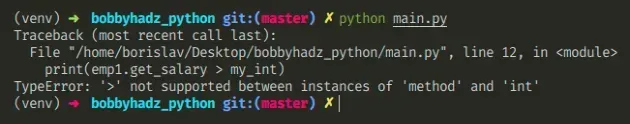Here is an example of how the error occurs.

main.py
```Copied!```class Employee():
def get_salary(self):
return 100

emp1 = Employee()

my_int = 10

# ⛔️ TypeError: '>' not supported between instances of 'method' and 'int'
print(emp1.get_salary > my_int) # 👈️ forgot to call method
``````
We forgot to call the method with parentheses, e.g. `emp.get_salary()`, so our code actually tries to compare a method and an integer.

## #Call the method to solve the error

To solve the error, make sure to call the method.

main.py
```Copied!```class Employee():
def get_salary(self):
return 100

emp1 = Employee()

my_int = 10

print(emp1.get_salary() > my_int)  # 👉️ True
``````

We used parentheses to invoke the method, so now we compare the return value of the method with an integer.

If your method takes arguments, make sure to pass them when calling it, e.g. `emp1.get_salary(10, 20)`.
main.py
```Copied!```class Employee():
def get_salary(self, num):
return 100 * num

emp1 = Employee()

my_int = 10

print(emp1.get_salary(2) > my_int)  # 👉️ True

print(emp1.get_salary(2))  # 👉️ 200
``````

We passed an argument in the call to the method

You might also get the error "TypeError: '>' not supported between instances of 'function' and 'int'".

Here is an example of how the error occurs.

main.py
```Copied!```def get_num():
return 50

my_int = 10

# ⛔️ TypeError: '>' not supported between instances of 'function' and 'int'
print(get_num > my_int) # 👈️ forgot to call method
``````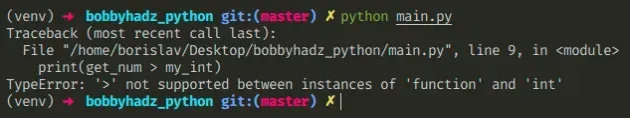We forgot to call the `get_num` function with parentheses.

To solve the error, make sure to call the function.

main.py
```Copied!```def get_num(num):
return 50 * num

my_int = 10

print(get_num(5) > my_int)  # 👉️ True

print(get_num(5))  # 👉️ 250
``````

We used parentheses to invoke the function, so now we compare the return value of the function with an integer.

If your function takes arguments, make sure to supply them.

I wrote a book in which I share everything I know about how to become a better, more efficient programmer.You can use the search field on my Home Page to filter through all of my articles.